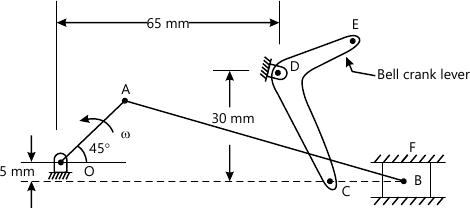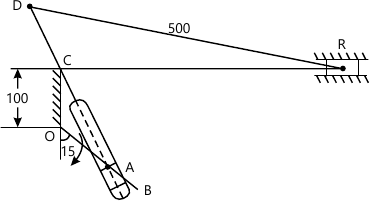MORE IN Theory of Machines - 1
MU Mechanical Engineering (Semester 4)
Theory of Machines - 1
May 2013
Total marks: --
Total time: --
INSTRUCTIONS
(1) Assume appropriate data and state your reasons
(2) Marks are given to the right of every question
(3) Draw neat diagrams wherever necessary

Explain any four:
1 (a) Classification of Kinematic Pairs.
5 M
1 (b) Rubbing velocity at a pin joint.
5 M
1 (c) Pantograph
5 M
1 (d) Work-Energy Principle
5 M
1 (e) Turning moment diagram.
5 M

2 (a) Draw neat sketch of Tchebicheffs mechanism and prove that the link lengths must be in the ratio of 1:2:2.5 for a point on coupler to trace an approximate straight line.
6 M
2 (b) In the mechanism shown in figure 1, the dimensions of various link are as follows: OA=25 mm, AB=80 mm, BC=20 mm, CD=33 mm, DE=20 mm. If the crank OA is inclined at 45° and revolves at a uniform speed of 120 r.p.m. in counter clockwise direction. Determine:
i) Angular velocities of links AB, BC and CD by Instantaneous Centre Method.
ii) Linear velocity of slider B by Relative Velocity Method.14 M

3 (a) Explain advantages and limitations of chain drive.
5 M
3 (b) In a Whiteworth quick return motion mechanism, OA is crank rotating at 30 rpm in clockwise direction. The dimensions of various links are OA=150 mm, OC=100 mm, CD=125 mm and DR=500 mm. Determine the acceleration of the sliding block R and the angular acceleration of the slotted lever CA.15 M

4 (a) Explain with the help of a neat sketch, how the limitation of Helical gear can be overcome in Herringbone gear.
5 M
4 (b) Explain any one inversion of Double Slider crank chain.
5 M
4 (c) The following data relate to a vertical cylinder four stroke IC engine-
i) Mass of reciprocating parts=8kg
iii) Mass of connecting rod=12kg
iv) Length of connecting rod=500 mm
v) Engine speed =300 rpm
vi) The distance between C.G. of connecting rod and big end of the bearing =200mm.
When connecting rod is suspended as pendulum from gudgen pin, it takes 11 seconds to complete oscillations.
Calculate:
i) The radius of gyration of connecting rod
ii) Torque on the crank shaft when crank has turned 60° and piston moves down.
10 M

5 (a) A capstan and rope are used in a railway goods yard for moving trucks. The capstan runs at 50 rpm. The rope from the line of trucks makes 2.75 turns round the capstan at a radius of 20 cm and the free end of the rope is pulled with a force f 15 kg. Determine the pull on the trucks, the HP taken by the trucks, the HP Taken by the trucks and the HP supplied by the capstone. (Take μ=0.25)
8 M
5 (b) A pair of involute spur gears is in mesh having 13 and 39 teeth on pinion and gear respectively. Both pinion and gear have addendum equal to one module which is 12 mm. Determine whether the gear interface with the pinion or not. If there is any interference, suggest suitable value of pressure angle which can avoid interference.
8 M
5 (c) State and prove Kennedy's Theorem.
4 M

6 (a) Derive an expression for correction couple to be applied to make two mass system Dynamically equivalent.
6 M
6 (b) In single Hooke's joint draw the polar diagram depicting the salient features of driven shaft speed.
4 M
6 (c) A punching machine has a capacity of producing 30 holes of 20 mm diameter per minute in a steel of 16mm thickness. The material of plate has a ultimate shear strength of 360 N/mm2. The actual punching operation lasts for a period of 36° rotation of the crank shaft. This crank shaft is powered by a flywheel through reduction gear having a ratio of 1:8 Take (i) mechanical efficiency=80% (ii) Speed fluctuation=10% (iii) Mean diameter of flywheel=0.75m (iv) Mass per unit length of flywheel material=7100 kg/m.
Determine:
(a) Power required
(b) Fluctuation of energy
(c) Cross Section of rim if the width to thickness ratio is 1.5
10 M

7 (a) Sketch the Davis steering gear mechanism and show that it satisfies the condition for correct steering. In spite of this, explain why in actual practice. Ackerman steering gear is preferred to the former?
10 M
7 (b) What is the significance of contact ratio in gear drives?
5 M
7 (c) Classify Belts.
5 M

More question papers from Theory of Machines - 1